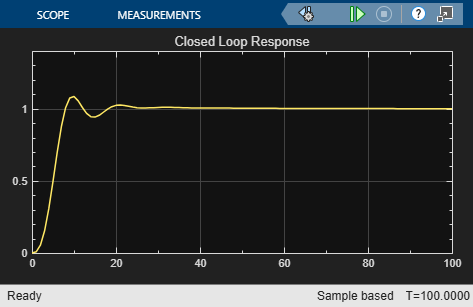# `lsqnonlin` with a Simulink® Model

This example shows how to tune the parameters of a Simulink model. The model, `optsim`, is included in the `optim`/`demos` folder of your MATLAB® installation. The model includes a nonlinear process plant modeled as a Simulink block diagram.Plant with Actuator Saturation

The plant is an under-damped third-order model with actuator limits. The actuator limits are a saturation limit and a slew rate limit. The actuator saturation limit cuts off input values greater than 2 units or less than –2 units. The slew rate limit of the actuator is 0.8 units/sec. The closed-loop response of the system to a step input is shown in Closed-Loop Response. You can see this response by opening the model (type `optsim` at the command line or click the model name), and selecting Run from the Simulation menu. The response plots to the scope.Closed-Loop Response

The problem is to design a feedback control loop that tracks a unit step input to the system. The closed-loop plant is entered in terms of the blocks where the plant and actuator are located in a hierarchical Subsystem block. A Scope block displays output trajectories during the design process.Closed-Loop Model

To solve this problem, minimize the error between the output and the input signal. (In contrast, in the example Using fminimax with a Simulink® Model, the solution involves minimizing the maximum value of the output.) The variables are the parameters of the Proportional Integral Derivative (PID) controller. If you only need to minimize the error at one time unit, you would have a scalar objective function. But the goal is to minimize the error for all time steps from 0 to 100, thus producing a multiobjective function (one function for each time step).

Use `lsqnonlin` to perform a least-squares fit on the tracking of the output. The tracking is performed by the function `tracklsq`, which is nested in `runtracklsq` at the end of this example. `tracklsq` returns the error signal `yout`, the output computed by calling `sim`, minus the input signal 1.

The function `runtracklsq` sets up all required values and then calls `lsqnonlin` with the objective function `tracklsq`. The variable `options` passed to `lsqnonlin` defines the criteria and display characteristics. The options specify to have no displayed output, to use the `'levenberg-marquardt'` algorithm, and the options give termination tolerances for the step and objective function on the order of 0.001.

To run the simulation in the model `optsim`, you must specify the variables `Kp`, `Ki`, `Kd`, `a1`, and `a2` (`a1` and `a2` are variables in the Plant block). `Kp`, `Ki`, and `Kd` are the variables to be optimized. The function `tracklsq` is nested inside `runtracklsq` so that the variables `a1` and `a2` are shared between the two functions. The variables `a1` and `a2` are initialized in `runtracklsq`.

The objective function `tracklsq` runs the simulation. You can run the simulation either in the base workspace or the current workspace, that is, the workspace of the function calling `sim`, which in this case is the workspace of `tracklsq`. In this example, the `SrcWorkspace` option is set to `'Current'` to tell `sim` to run the simulation in the current workspace. `runtracklsq` runs the simulation to 100 seconds.

When the simulation is complete, `runtracklsq` creates the `myobj` object in the current workspace (that is, the workspace of `tracklsq`). The Outport block in the block diagram model puts the `yout` field of the object into the current workspace at the end of the simulation.

When you run `runtracklsq`, the optimization gives the solution for the proportional, integral, and derivative (`Kp`, `Ki`, `Kd`) gains of the controller.

`[Kp, Ki, Kd] = runtracklsq`
```Kp = 3.1330 ```
```Ki = 0.1465 ```
```Kd = 14.3918 ```

The scope shows the optimized closed-loop step response.Closed-Loop Response After `lsqnonlin`

Note: The call to `sim` results in a call to one of the Simulink ordinary differential equation (ODE) solvers. You need to choose which type of solver to use. From the optimization point of view, a fixed-step ODE solver is the best choice if it is sufficient to solve the ODE. However, in the case of a stiff system, a variable-step ODE method might be required to solve the ODE.

The numerical solution produced by a variable-step solver, however, is not a smooth function of parameters, because of step-size control mechanisms. This lack of smoothness can prevent an optimization routine from converging. The lack of smoothness is not an issue when you use a fixed-step solver. (For a further explanation, see .)

Simulink Design Optimization™ software is recommended for solving multiobjective optimization problems in conjunction with Simulink variable-step solvers. This software provides a special numeric gradient computation that works with Simulink and avoids introducing a problem of lack of smoothness.

### Helper Function

The following code creates the `runtracklsq` helper function.

```function [Kp,Ki,Kd] = runtracklsq % RUNTRACKLSQ demonstrates using LSQNONLIN with Simulink. mdl = 'optsim'; open_system(mdl) % Load the model in = Simulink.SimulationInput(mdl); % Create simulation input object in = in.setModelParameter('StopTime','100'); % Stop time 100 pid0 = [0.63 0.0504 1.9688]; % Initial gain values a1 = 3; a2 = 43; % Initialize model plant variables options = optimoptions(@lsqnonlin,'Algorithm','levenberg-marquardt',... 'Display','off','StepTolerance',0.001,'OptimalityTolerance',0.001); % Optimize the gains set_param(mdl,'FastRestart','on'); % Fast restart pid = lsqnonlin(@tracklsq,pid0,[],[],options); set_param(mdl,'FastRestart','off'); % Return the gains Kp = pid(1); Ki = pid(2); Kd = pid(3); function F = tracklsq(pid) % Track the output of optsim to a signal of 1 % Set the simulation input object parameters in = in.setVariable('Kp',pid(1),'Workspace',mdl); in = in.setVariable('Ki',pid(2),'Workspace',mdl); in = in.setVariable('Kd',pid(3),'Workspace',mdl); % Simulate out = sim(in); F = out.get('yout') - 1; end end```